# Area of a triangle formed by three tangent lines of three tangent circles

#### yourplayin

##### New member
Find the area of the triangle formed by the three tangent lines of three tangent circles, that are parallel to the segments connecting radii pairs and tangent to the third circle, within the area bounded by the three circles. Assume the radii are 5, 7 and 9 feet.

I have attached my work. Here is my question. I have calculated the area of the region between the circles. Can I some how use this area to find the triangle that lies inside the shadded area?

#### Attachments

• 1.1 MB Views: 18

#### pka

##### Elite Member
Find the area of the triangle formed by the three tangent lines of three tangent circles, that are parallel to the segments connecting radii pairs and tangent to the third circle, within the area bounded by the three circles. Assume the radii are 5, 7 and 9 feet.
I have attached my work. Here is my question. I have calculated the area of the region between the circles. Can I some how use this area to find the triangle that lies inside the shaded area?
To yourplayin, Question why can you not simply state the conditions of the questions and ask the real questions?
I have downloded (with great great trouble) the PdF files. Trying to read the files in next to impossible for me.
A simple posting of the actual statement of the original question is so much more efficient.

•MarkFL

#### yourplayin

##### New member
Pka,

I see that you are an elite member, so I say this with the most respect possible. I’m searching for a better understanding of math. I’m not asking to be criticized. If I didn’t not follow the rules then please advise me on what I shall do the next time I decide to ask a question. But to be upset about the way I made my post is the exact reason that people don’t ask for help. Thank you and have a great day.

#### MarkFL

##### Super Moderator
Staff member
Most people helping with math would rather not download a file, it's much better to attach any images to your post, and display it within your post. It's not so much a criticism but more of a suggestion on how to get help in a more timely manner.

Can you give the original and complete problem statement?

•pka

#### yourplayin

##### New member
Most people helping with math would rather not download a file, it's much better to attach any images to your post, and display it within your post. It's not so much a criticism but more of a suggestion on how to get help in a more timely manner.

Can you give the original and complete problem statement?

Find the area of the triangle formed by the three tangent lines of three tangent circles, that are parallel to the segments connecting radii pairs and tangent to the third circle, within the area bounded by the three circles. Assume the radii are 5, 7 and 9 feet.

My question: I’ve found the area between the tangent circles but I’m not really sure this is useful. I’m looking for guidance on how to proceed .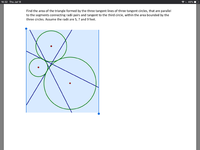#### MarkFL

##### Super Moderator
Staff member
Okay, you are off to a good start finding the area of the triangle whose vertices lie at the centers of the circles, because the triangle whose area we are to find is similar to that, since it must have the same 3 interior angles.

What I would try next is to find the length of one of the sides of the smaller triangle, and use the fact that the area of the smaller triangle is proportional to the square of this length, and then use the fact that the larger triangle is proportional to the square of the corresponding side, and the constant of proportionality must be the same since the two triangles are similar.

I would use coordinate geometry to find the length of one of the sides of the smaller triangle. Here's a graph to get you started: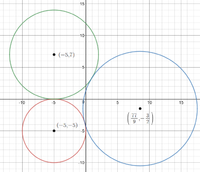#### yourplayin

##### New member
Okay, you are off to a good start finding the area of the triangle whose vertices lie at the centers of the circles, because the triangle whose area we are to find is similar to that, since it must have the same 3 interior angles.

What I would try next is to find the length of one of the sides of the smaller triangle, and use the fact that the area of the smaller triangle is proportional to the square of this length, and then use the fact that the larger triangle is proportional to the square of the corresponding side, and the constant of proportionality must be the same since the two triangles are similar.

I would use coordinate geometry to find the length of one of the sides of the smaller triangle. Here's a graph to get you started:

View attachment 12962
If I found the slip of the tangent line that makes up the base of the triangle and use the fact that a perpendicular line makes a 90 degree angle, then I could use an inverse trig function to find the second length of another tangent line. Is this correct? Also thank you for the grid. It really ties it together for me.

#### MarkFL

##### Super Moderator
Staff member
I would next go about finding the tangent lines.

We know the line tangent to the largest circle is vertical, and so it is:

$$\displaystyle x=-\frac{4}{9}$$

For the line tangent to the circle of radius 7, we know its slope must be:

$$\displaystyle m=\frac{-\dfrac{3}{2}+5}{\dfrac{77}{9}+5}=\frac{63}{244}$$

To find a point on this line, we can use the tangent point, because we know the line through this point and the circle to which it is tangent, must be perpendicular to the tangent line.

$$\displaystyle \frac{y_1-7}{x_1+5}=-\frac{244}{63}$$

We also know this point is on the circle:

$$\displaystyle (x_1+5)^2+(y_1-7)^2=7^2$$

Solving this system, and choosing the appropriate solution, we obtain:

$$\displaystyle (x_1,y_1)=\left(-5+\frac{441}{\sqrt{63505}},7-\frac{1708}{\sqrt{63505}}\right)$$

Thus, the line tangent to the circle of radius 7 is:

$$\displaystyle y=\frac{63}{244}\left(x+5-\frac{441}{\sqrt{63505}}\right)+7-\frac{1708}{\sqrt{63505}}$$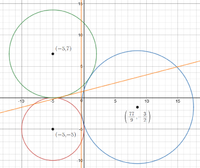Can you find the equation of the line tangent to the smallest circle?

Last edited:

#### yourplayin

##### New member
I would next go about finding the tangent lines.

We know the line tangent to the largest circle is vertical, and so it is:

$$\displaystyle x=-\frac{4}{9}$$

For the line tangent to the circle of radius 7, we know its slope must be:

$$\displaystyle m=\frac{-\dfrac{3}{2}+5}{\dfrac{77}{9}+5}=\frac{63}{244}$$

To find a point on this line, we can use the tangent point, because we know the line through this point and the circle to which it is tangent, must be perpendicular to the tangent line.

$$\displaystyle \frac{y_1-7}{x_1+5}=-\frac{244}{63}$$

We also know this point is on the circle:

$$\displaystyle (x_1+5)^2+(y_1-7)^2=7^2$$

Solving this system, and choosing the appropriate solution, we obtain:

$$\displaystyle (x_1,y_1)=\left(-5+\frac{441}{\sqrt{63505}},7-\frac{1708}{\sqrt{63505}}\right)$$

Thus, the line tangent to the circle of radius 7 is:

$$\displaystyle y=\frac{63}{244}\left(x+5-\frac{441}{\sqrt{63505}}\right)+7-\frac{1708}{\sqrt{63505}}$$

View attachment 12964

Can you find the equation of the line tangent to the smallest circle?
I will work this tomorrow and post my answer. Again thank you for the help.

•MarkFL

#### MarkFL

##### Super Moderator
Staff member
Something tells me there's a much easier way to work this, but I'll be dogged if I can see it right now.#### Subhotosh Khan

##### Super Moderator
Staff member
Find the area of the triangle formed by the three tangent lines of three tangent circles, that are parallel to the segments connecting radii pairs and tangent to the third circle, within the area bounded by the three circles. Assume the radii are 5, 7 and 9 feet.

I have attached my work. Here is my question. I have calculated the area of the region between the circles. Can I some how use this area to find the triangle that lies inside the shadded area?
I don't see any shaded area!

#### MarkFL

##### Super Moderator
Staff member
To follow up, the slope of the line tangent to the smallest circle must be:

$$\displaystyle m=\frac{-\dfrac{3}{2}-7}{\dfrac{77}{9}+5}=-\frac{153}{244}$$

To find the tangent point, we may use:

$$\displaystyle \frac{y_1+5}{x_1+5}=\frac{244}{153}$$

$$\displaystyle (x_1+5)^2+(y_1+5)^2=5^2$$

Solving this system, we obtain:

$$\displaystyle (x_1,y_1)=\left(153\sqrt{\frac{5}{16589}}-5,244\sqrt{\frac{5}{16589}}-5\right)$$

And so the equation of the tangent line is:

$$\displaystyle y=-\frac{153}{244}\left(x+5-153\sqrt{\frac{5}{16589}}\right)+244\sqrt{\frac{5}{16589}}-5$$

Here is a plot of everything we have so far: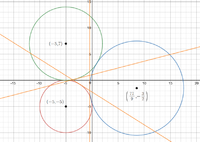Here is a plot with just the tangent lines, and the triangular area shaded, and two of the vertices labeled.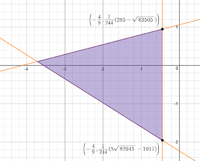Can you proceed, using the method involving similarity I outlined in my first post?

#### yourplayin

##### New member
Yes. I’ve been working on it and for some reason it didn’t click until just now. Thank you.

#### yourplayin

##### New member
What program are you using to make the diagram?

#### MarkFL

##### Super Moderator
Staff member
What program are you using to make the diagram?
I am using Desmos for the diagrams.I'll finish the problem tomorrow, to give you a chance to work on it first.

#### yourplayin

##### New member
We are looking for the area of the triangle, which is A=1/2(B*H). Can I not just add the points along the y axis and then multiply by the point 3.722 ? I feel as though this is too simple.

#### yourplayin

##### New member
Okay I believe I've found it. I used coordinate geometry to locate the area of the triangle. using the formula:
area = (1/2)|Ax(By-Cy)+Bx(Cy-Ay)+Cx(Ay-By)| for a value of 4.75638. Is this correct?

#### MarkFL

##### Super Moderator
Staff member
Okay, let's finish this up...let's call the area of the triangle whose vertices are at the centers of the 3 circles $$A_V$$. We then have:

$$\displaystyle A_V=\frac{1}{2}(7-(-5))\left(\frac{77}{9}-(-5)\right)=\frac{244}{3}$$

We may also state:

$$\displaystyle A_V=k(12)^2\implies k=\frac{61}{108}$$

Now, for the triangle whose area $$A$$ we are instructed to find, we may write:

$$\displaystyle A=k\left(\frac{1}{244}\left(3912-7\sqrt{63505}-5\sqrt{82945}\right)\right)^2$$

Since the two triangles are similar, the value of $$k$$ is the same, thus:

$$\displaystyle A=\frac{\left(3912-7\sqrt{63505}-5\sqrt{82945}\right)^2}{105408}\approx4.7551612998113334888567878747$$

Okay I believe I've found it. I used coordinate geometry to locate the area of the triangle. using the formula:
area = (1/2)|Ax(By-Cy)+Bx(Cy-Ay)+Cx(Ay-By)| for a value of 4.75638. Is this correct?
Our result are quite similar...did you do any intermediary rounding?

#### yourplayin

##### New member
Okay, let's finish this up...let's call the area of the triangle whose vertices are at the centers of the 3 circles $$A_V$$. We then have:

$$\displaystyle A_V=\frac{1}{2}(7-(-5))\left(\frac{77}{9}-(-5)\right)=\frac{244}{3}$$

We may also state:

$$\displaystyle A_V=k(12)^2\implies k=\frac{61}{108}$$

Now, for the triangle whose area $$A$$ we are instructed to find, we may write:

$$\displaystyle A=k\left(\frac{1}{244}\left(3912-7\sqrt{63505}-5\sqrt{82945}\right)\right)^2$$

Since the two triangles are similar, the value of $$k$$ is the same, thus:

$$\displaystyle A=\frac{\left(3912-7\sqrt{63505}-5\sqrt{82945}\right)^2}{105408}\approx4.7551612998113334888567878747$$

Our result are quite similar...did you do any intermediary rounding?
My calculator may have rounded it for me. I will adjust out the decimal places for the rounding.

#### MarkFL

##### Super Moderator
Staff member
I unfortunately made an error in my placement of the largest circle, so I am going to work the problem again, from start to finish, using the correct location.

Let's begin by placing the two smaller circles in the plane as follows: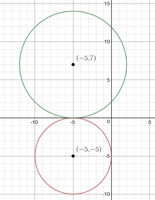Now, the center of the largest circle must satisfy the nonlinear system:

$$\displaystyle (x+5)^2+(y+5)^2=14^2$$

$$\displaystyle (x+5)^2+(y-7)^2=16^2$$

Solving this system, and taking the solution which places the largest circle to the right of the others, we obtain:

$$\displaystyle (x,y)=\left(\frac{7}{2}\sqrt{15}-5,-\frac{3}{2}\right)$$

And so we now place the largest circle in the plane: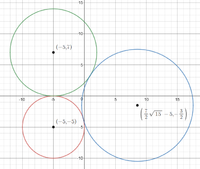The vertical tangent line (tangent to the largest circle) will be:

$$\displaystyle x=\frac{7}{2}\sqrt{15}-14$$

The line tangent to the circle of radius 7 will have a slope of:

$$\displaystyle m=\frac{-\dfrac{3}{2}+5}{\dfrac{7}{2}\sqrt{15}-5-(-5)}=\frac{1}{\sqrt{15}}$$

The tangent point is obtained through:

$$\displaystyle \frac{7-y_1}{-5-x_1}=-\sqrt{15}$$

$$\displaystyle (x_1+5)^2+(y_1-7)^2=7^2$$

This gives us the tangent point:

$$\displaystyle \left(x_1,y_1\right)=\left(-\frac{13}{4},\frac{7}{4}(4-\sqrt{15})\right)$$

And so this tangent line has the equation:

$$\displaystyle y=\frac{1}{\sqrt{15}}x+7-\frac{23}{\sqrt{15}}$$

For the line tangent to the smallest circle, it's slope is:

$$\displaystyle m=\frac{-\dfrac{3}{2}-7}{\dfrac{7}{2}\sqrt{15}-5-(-5)}=-\frac{17}{7\sqrt{15}}$$

The tangent point is obtained through:

$$\displaystyle \frac{-5-y_1}{-5-x_1}=\frac{7\sqrt{15}}{17}$$

$$\displaystyle (x_1+5)^2+(y_1+5)^2=5^2$$

This gives us the tangent point:

$$\displaystyle \left(x_1,y_1\right)=\left(-\frac{75}{32},\frac{35}{32}\sqrt{15}-5\right)$$

And so this tangent line has the equation:

$$\displaystyle y=-\frac{17}{7\sqrt{15}}x+\frac{817}{224}\sqrt{\frac{3}{5}}-5$$

Here is a plot including the three tangent lines: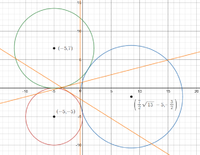We then find that the length of the vertical side of the triangle whose area we are finding is:

$$\displaystyle \left(\frac{21}{2}-\frac{37}{\sqrt{15}}\right)-\left(\frac{10067}{224\sqrt{15}}-\frac{27}{2}\right)=\frac{1}{672}\left(16128-3671\sqrt{15}\right)$$

The area of the triangle whose vertices are at the centers of the circles is:

$$\displaystyle A_V=\frac{1}{2}(7-(-5))\left(\frac{7}{2}\sqrt{15}-5-(-5)\right)=21\sqrt{15}$$

Also:

$$\displaystyle A_V=k(12)^2\implies k=\frac{7\sqrt{15}}{48}$$

Hence, the area we want is:

$$\displaystyle A=\frac{7\sqrt{15}}{48}\left(\frac{1}{672}\left(16128-3671\sqrt{15}\right)\right)^2=\frac{154085333\sqrt{15}-592058880}{1032192}\approx4.56412$$

I sure hope I didn't make any other errors!!